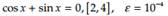Create an Account

Home / Questions / Modify the user-defined function Bisection Section 3 2 so that the table is not generated...

Modify the user-defined function Bisection Section 3 2 so that the table is not generated and the outputs are the approximate root and the number of iterations needed for the tolerance to be met

Modify the user-defined function Bisection (Section 3.2) so that the table is not generated and the outputs are the approximate root and the number of iterations needed for the tolerance to be met. All other parameters, including default values, are to remain unchanged. Save this function as Bisection_New. Apply Bisection_New to the equation in Problem 1, using the given tolerance:Problem 1

Using bisection method, generate the first four midpoints and intervals (besides the original interval given) containing the root.

cos x + sin x = 0, [2,4]

Jul 22 2020 View more View LessSubscribe To Get Solution